Question

# Two very long, straight, parallel wires carry currents that are directed perpendicular to the page as...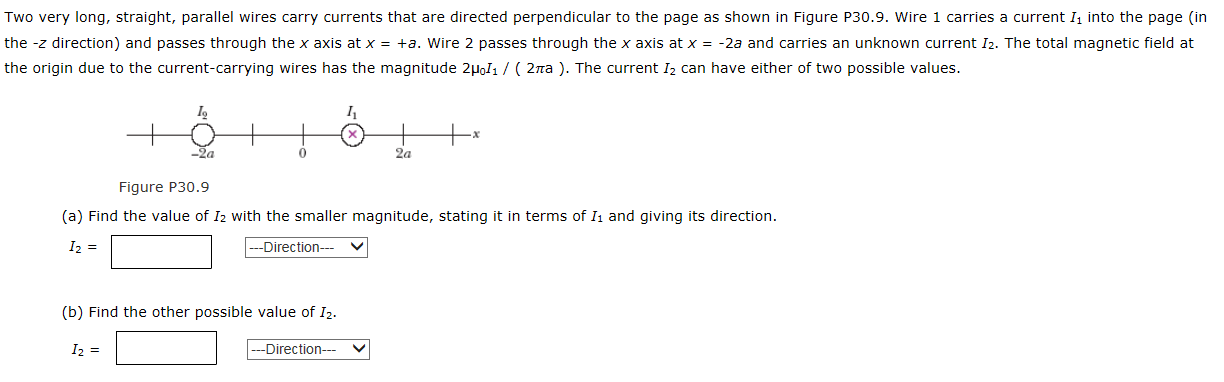Two very long, straight, parallel wires carry currents that are directed perpendicular to the page as shown in Figure P30.9. Wire 1 carries a current 1. into the page (in the -z direction) and passes through the x axis at x = -2a. Wire 2 passes through the x axis at x = -+a and carries an unknown current 12. The total magnetic field at the origin due to the current-carrying wires has the magnitude 2PoIi / ( 2 pira ). The current I2 can have either of two possible values.Figure P30.9 (a) Find the value of Iz with the smaller magnitude, stating it in terms of Ii and giving its direction. I2 = (b) Find the other possible value of I2. I2 =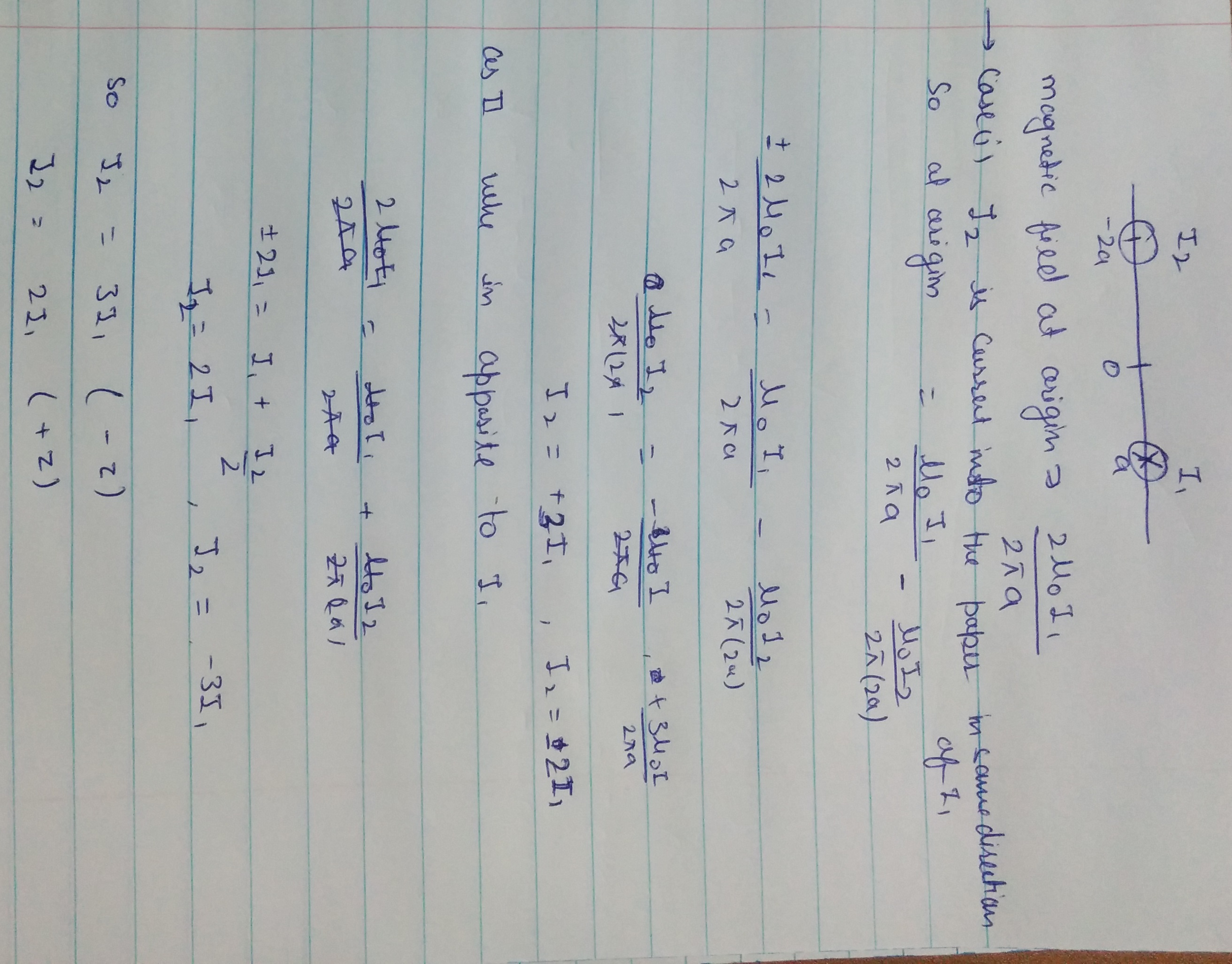#### Earn Coins

Coins can be redeemed for fabulous gifts.

Similar Homework Help Questions
• ### The figure below shows a cross-section of two long, straight, parallel wires, where the currents are flowing perpendicul...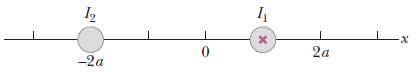The figure below shows a cross-section of two long, straight, parallel wires, where the currents are flowing perpendicular to the page. Wire 1 carries a current I1 directed into the page (in the −z direction) and passes through the x-axis at x = +a. Wire 2 passes through the x-axis at x = −2a and carries an unknown current I2. The total magnetic field at the origin due to the current-carrying wires has the magnitude 2μ0I1 (2πa) . Note that...

• ### The figure below shows two long, parallel wires incross-section, and the currents they carry are...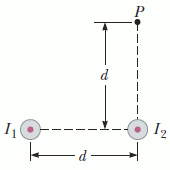The figure below shows two long, parallel wires in cross-section, and the currents they carry are directed out of the page. The left wire carries a current ofI1 = 2.96 A,and the right carries a current ofI2 = 3.45 A.The two wires are a distanced = 20.0 cmapart. Point P is the same distance d above the right wire.Assume the +x-direction is to the right, and the +y-direction is upward.A cross-sectional end view of two parallel wires carrying current out...

• ### Three long straight wires are parallel to each other and carry currents directed into or out...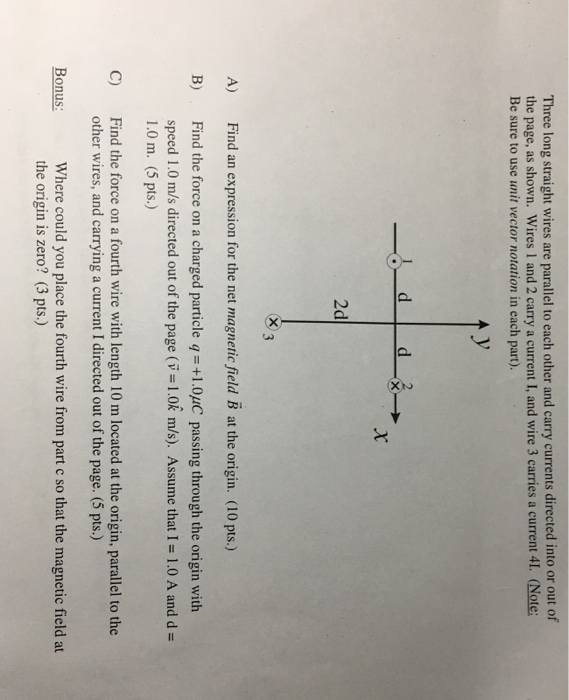Three long straight wires are parallel to each other and carry currents directed into or out of the page, as shown. Wires 1 and 2 carry a current I, and wire 3 carries a current 41. (Note: Be sure to use unit vector notation in each part). 2d indn rssio for th net magnaie fald B ) B) Find the force on a charged particle q-+1.0uC passing through the origin with speed 1.0 m/s directed out of the page (v-1.0k...

• ### Problem 1 Three long straight wires are parallel to each other and carry currents directed into...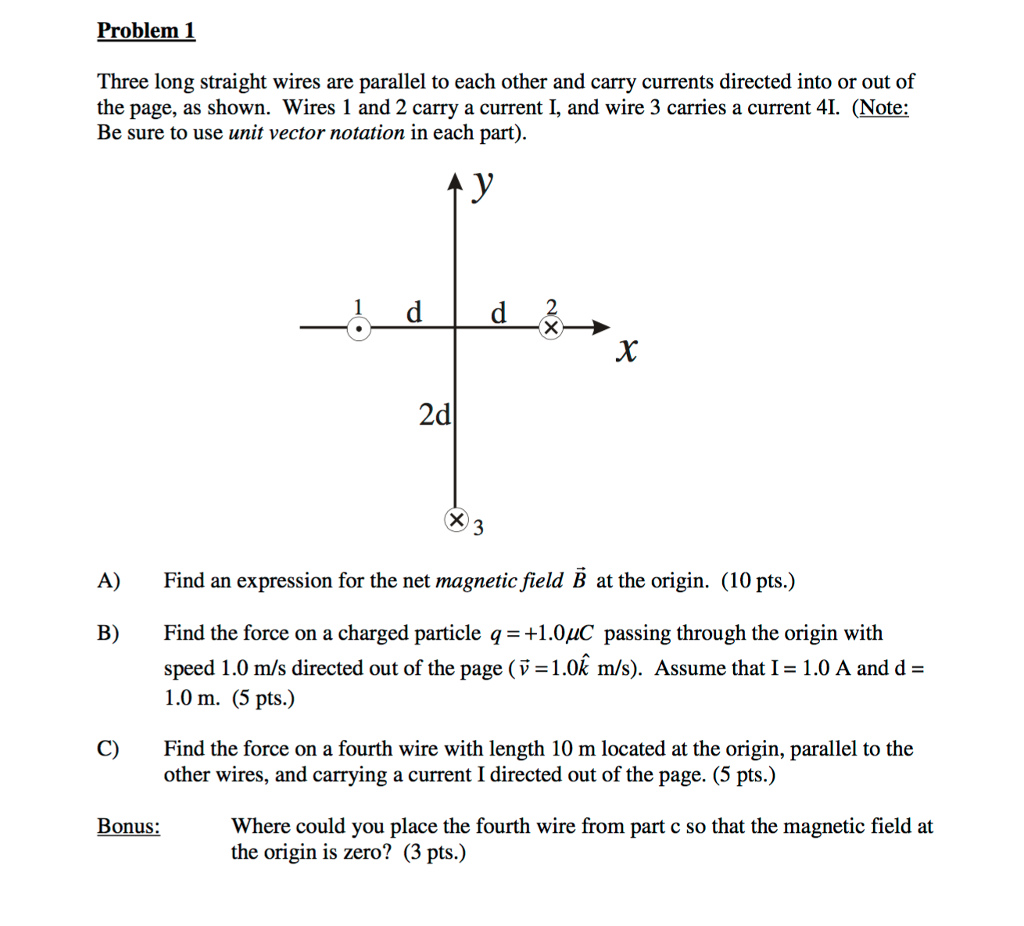Problem 1 Three long straight wires are parallel to each other and carry currents directed into or out of the page, as shown. Wires 1 and 2 carry a current I, and wire 3 carries a current 4. (Note: Be sure to use unit vector notation in each part) 2 2d A) Find an expression for the net magnetic field B at the origin. (10 pts.) B) Find the force on a charged particle q1.0uC passing through the origin with...

• ### Question 20 (7 points) Two long straight wires carry currents perpendicular to the xy-plane. One wire...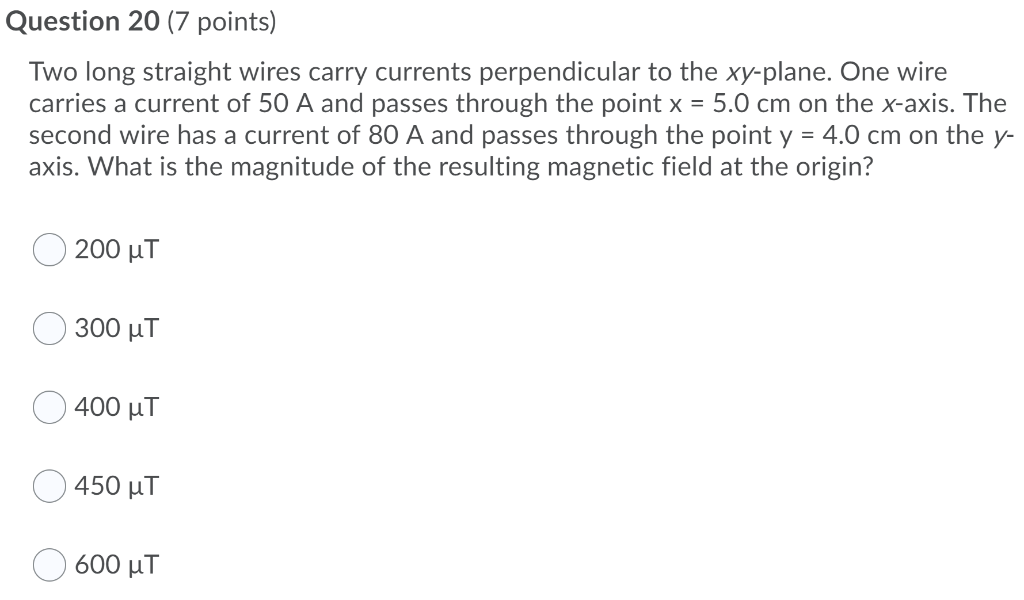Question 20 (7 points) Two long straight wires carry currents perpendicular to the xy-plane. One wire carries a current of 50 A and passes through the point x = 5.0 cm on the x-axis. The second wire has a current of 80 A and passes through the point y = 4.0 cm on the y- axis. What is the magnitude of the resulting magnetic field at the origin? 200 ut 300 uT 400 uT 450 uT 600 uT

• ### Four long straight parallel wires are oriented perpendicular to the x-y plane and carry currents I1,...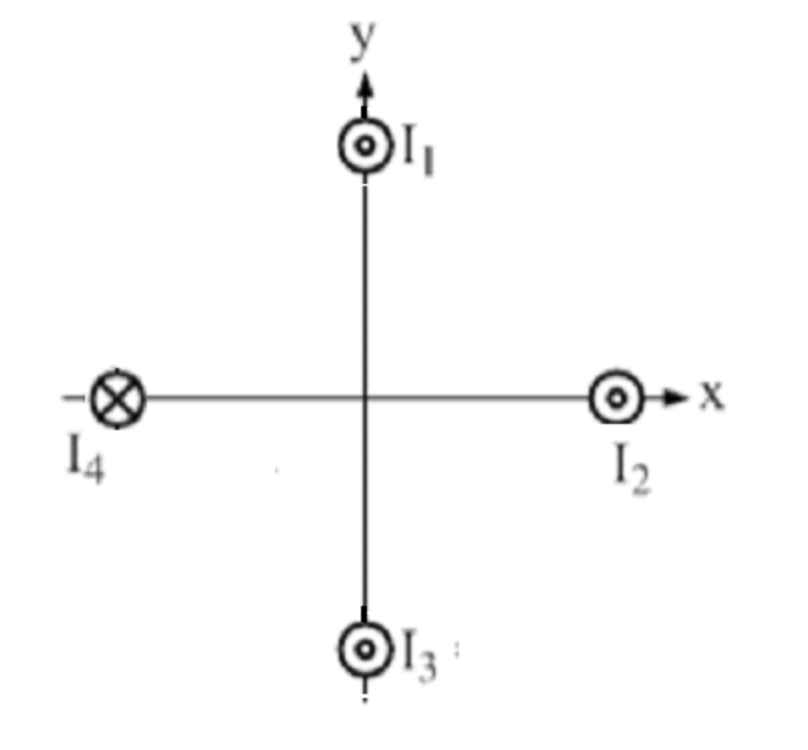Four long straight parallel wires are oriented perpendicular to the x-y plane and carry currents I1, I2, I3 and I4. The magnitudes of the currents are all identical and equal to 8.475 A. Current I4 is into the x-y plane (i.e. into the screen), whereas each of the other three currents are all out of the x-y plane(i.e. out of the screen) as shown below. Each of the wires is a distance of 6.105 cm from the origin as measured...

• ### Four long straight parallel wires are oriented perpendicular to the x-y plane and carry currents I1,...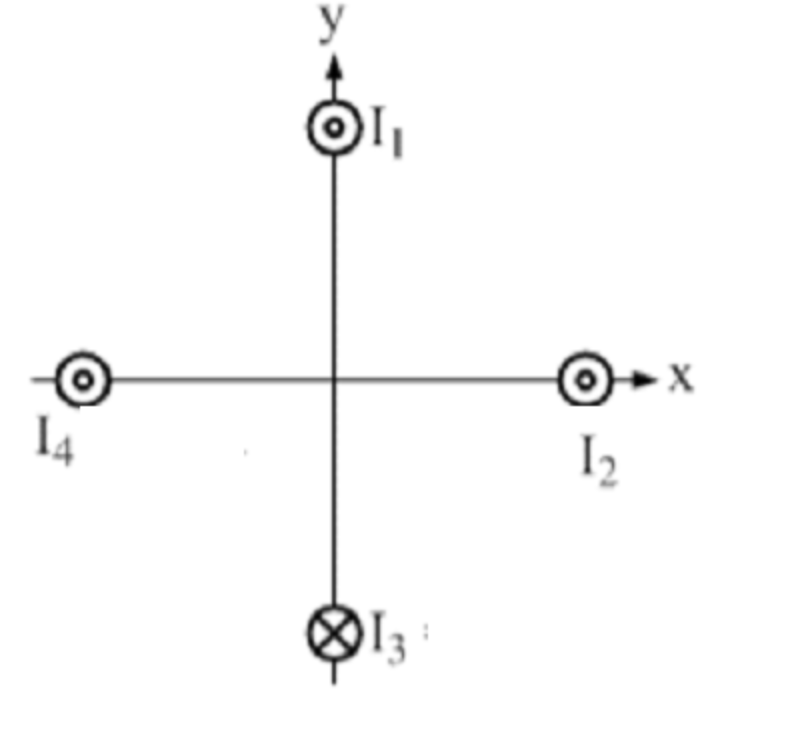Four long straight parallel wires are oriented perpendicular to the x-y plane and carry currents I1, I2, I3 and I4. The magnitudes of the currents are all identical and equal to 4.015 A. Current I3 is into the x-y plane (i.e. into the screen), whereas each of the other three currents are all out of the x-y plane(i.e. out of the screen) as shown below. Each of the wires is a distance of 2.987 cm from the origin as measured...

• ### Two infinitely long, straight parallel wires perpendicular to the plane of the page carry currents of...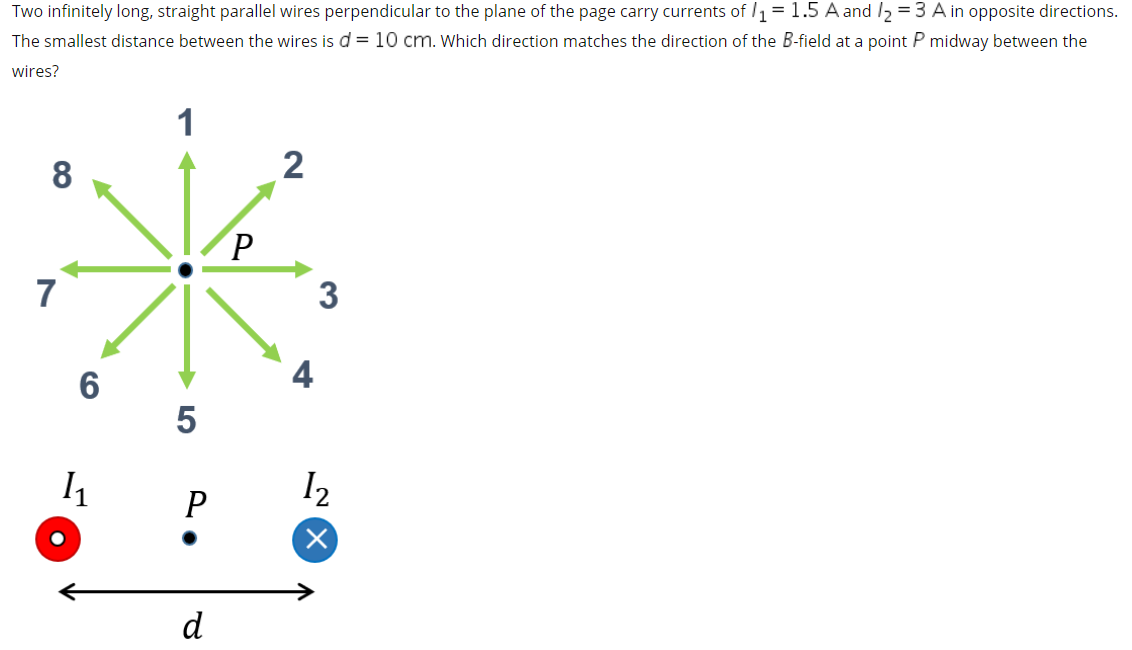Two infinitely long, straight parallel wires perpendicular to the plane of the page carry currents of l1 = 1.5 A and I2 = 3 A in opposite directions. The smallest distance between the wires is d = 10 cm. Which direction matches the direction of the B-field at a point P midway between the wires?

• ### 2. Two very long wires are oriented parallel to the y axis and carry identical but...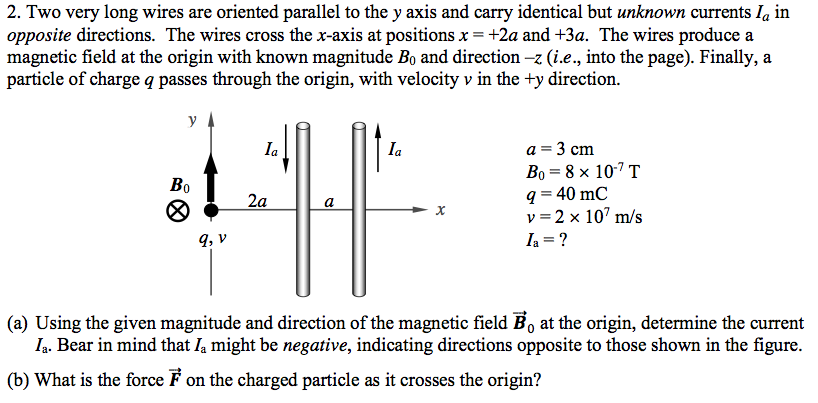2. Two very long wires are oriented parallel to the y axis and carry identical but unknown currents Ia inopposite directions. The wires cross the x-axis at positions x = +2a and +3a. The wires produce amagnetic field at the origin with known magnitude B0 and direction -z (i.e., into the page). Finally, aparticle of charge q passes through the origin, with velocity y in the +y direction.(a) Using the given magnitude and direction of the magnetic field ii at...

• ### Two long, parallel wires carry currents of I1 = 2.85 A and I2 = 5.45 A...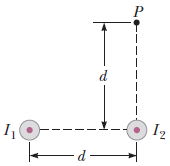Two long, parallel wires carry currents of I1 = 2.85 A and I2 = 5.45 A in the directions indicated in the figure below, where d = 19.5 cm. (Take the positive x direction to be to the right.) (a) Find the magnitude and direction of the magnetic field at a point midway between the wires. magnitude______  μT direction________ ° counterclockwise from the +x axis (b) Find the magnitude and direction of the magnetic field at point P, located d =...